Filters
Size

# COLOUR TSHIRTS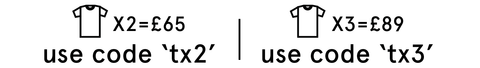Filters

Selected Filters• XS
• S
• M
• L
• XL
• XXL
• Select Size• XS
• S
• M
• L
• XL
• XXL
• Select Size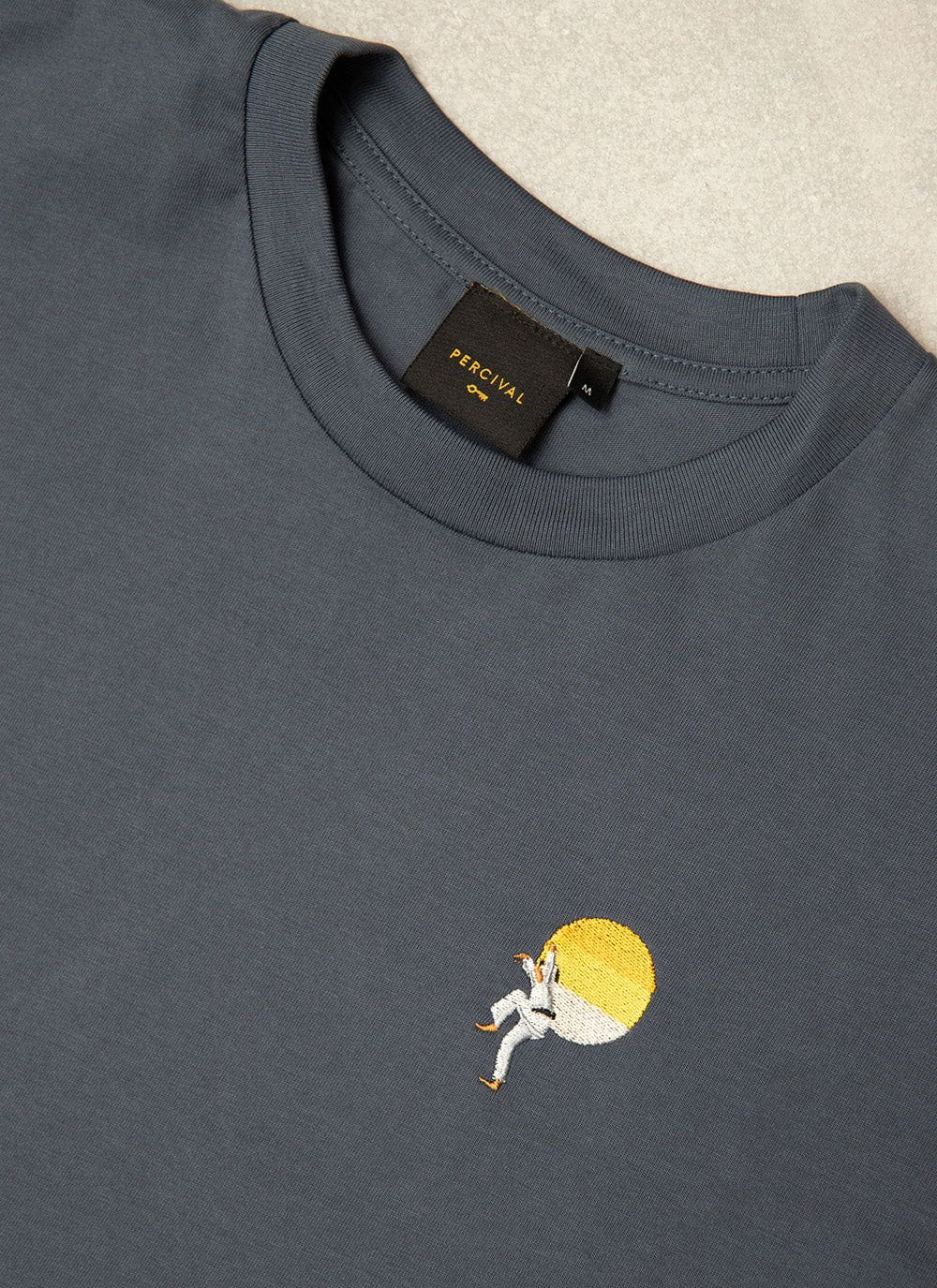• XS
• S
• M
• L
• XL
• XXL
• Select Size
Running Low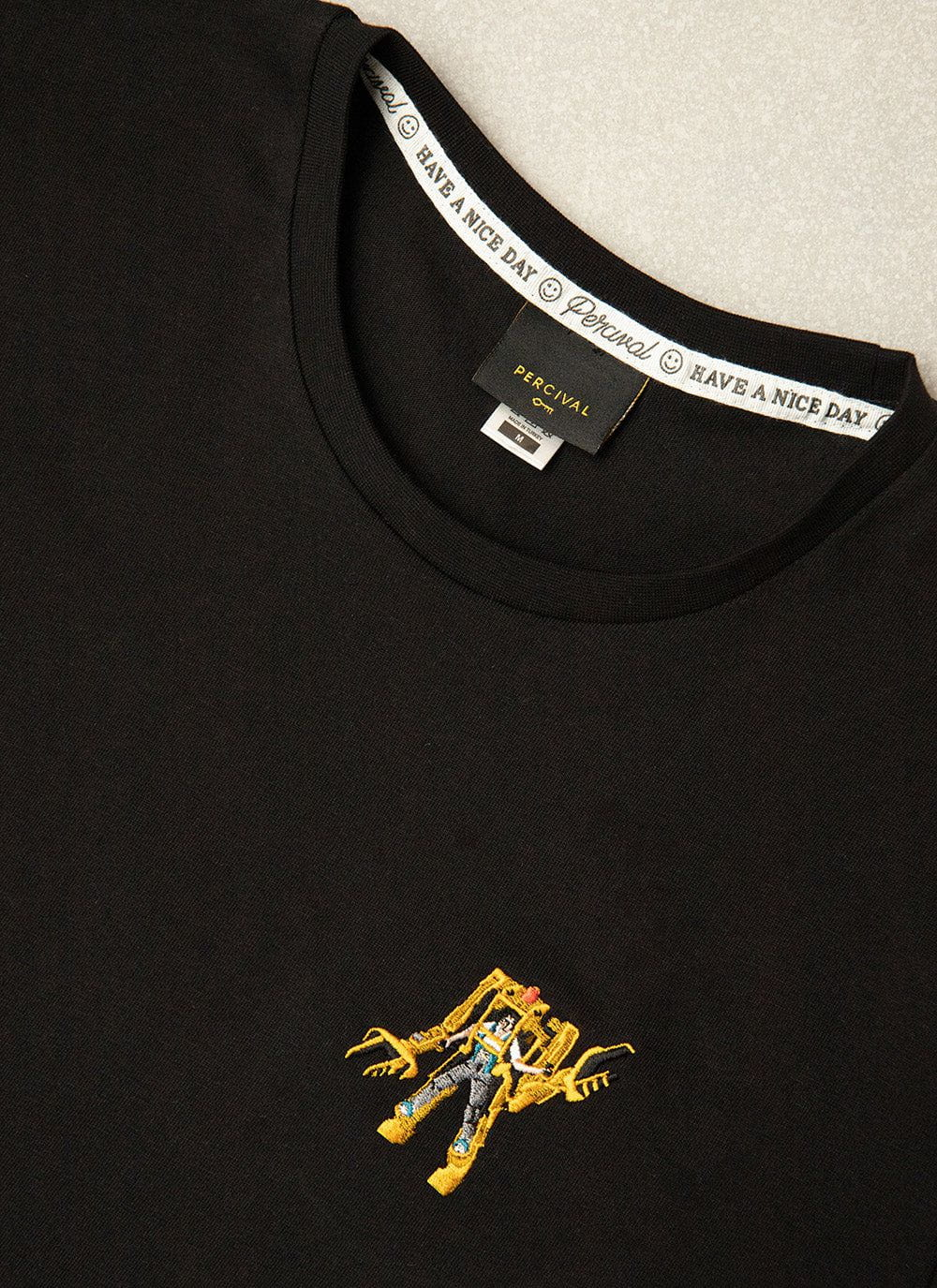• XS
• S
• M
• L
• XL
• XXL
• Select Size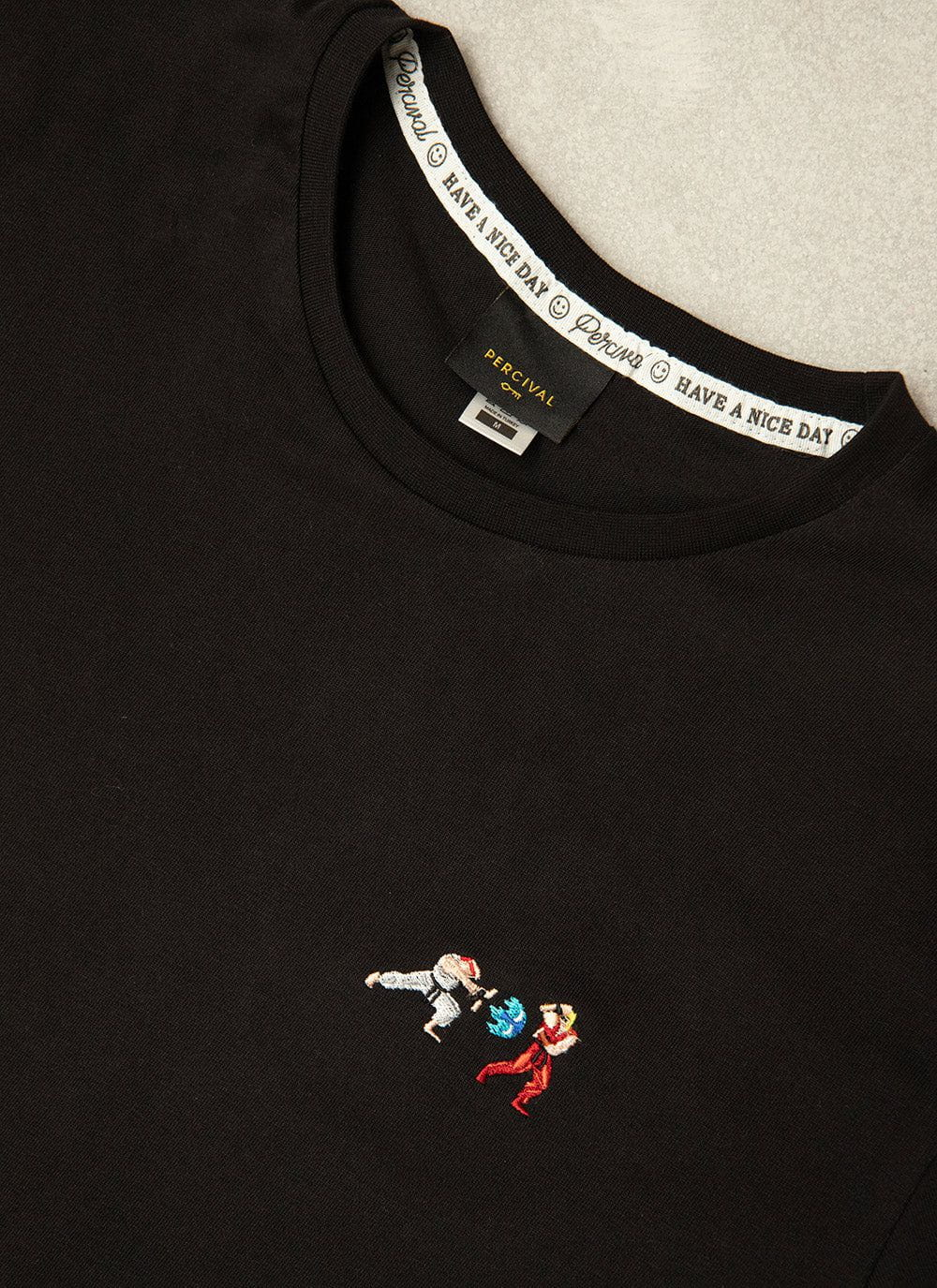• XS
• S
• M
• L
• XL
• XXL
• Select Size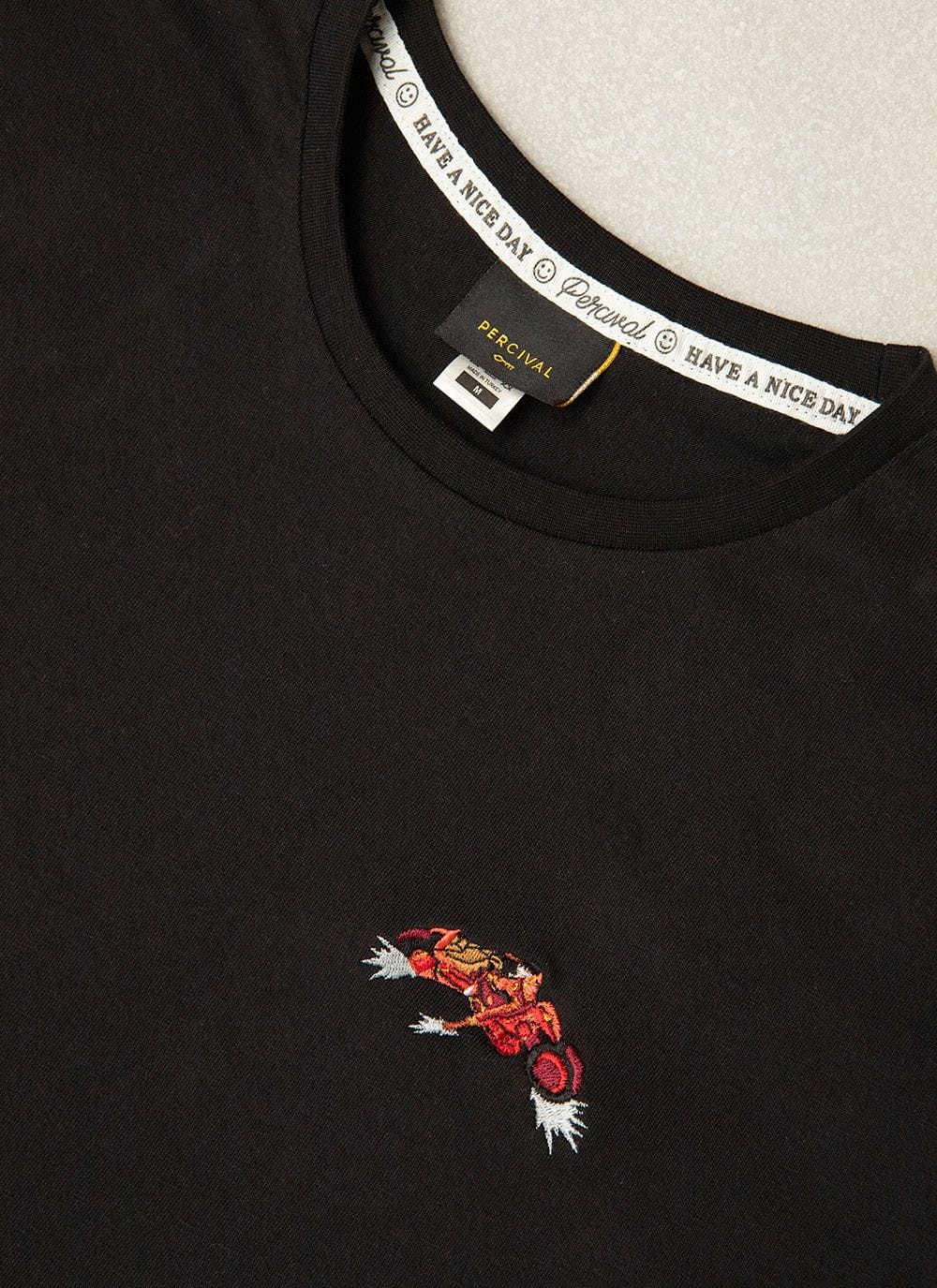• XS
• S
• M
• L
• XL
• XXL
• Select Size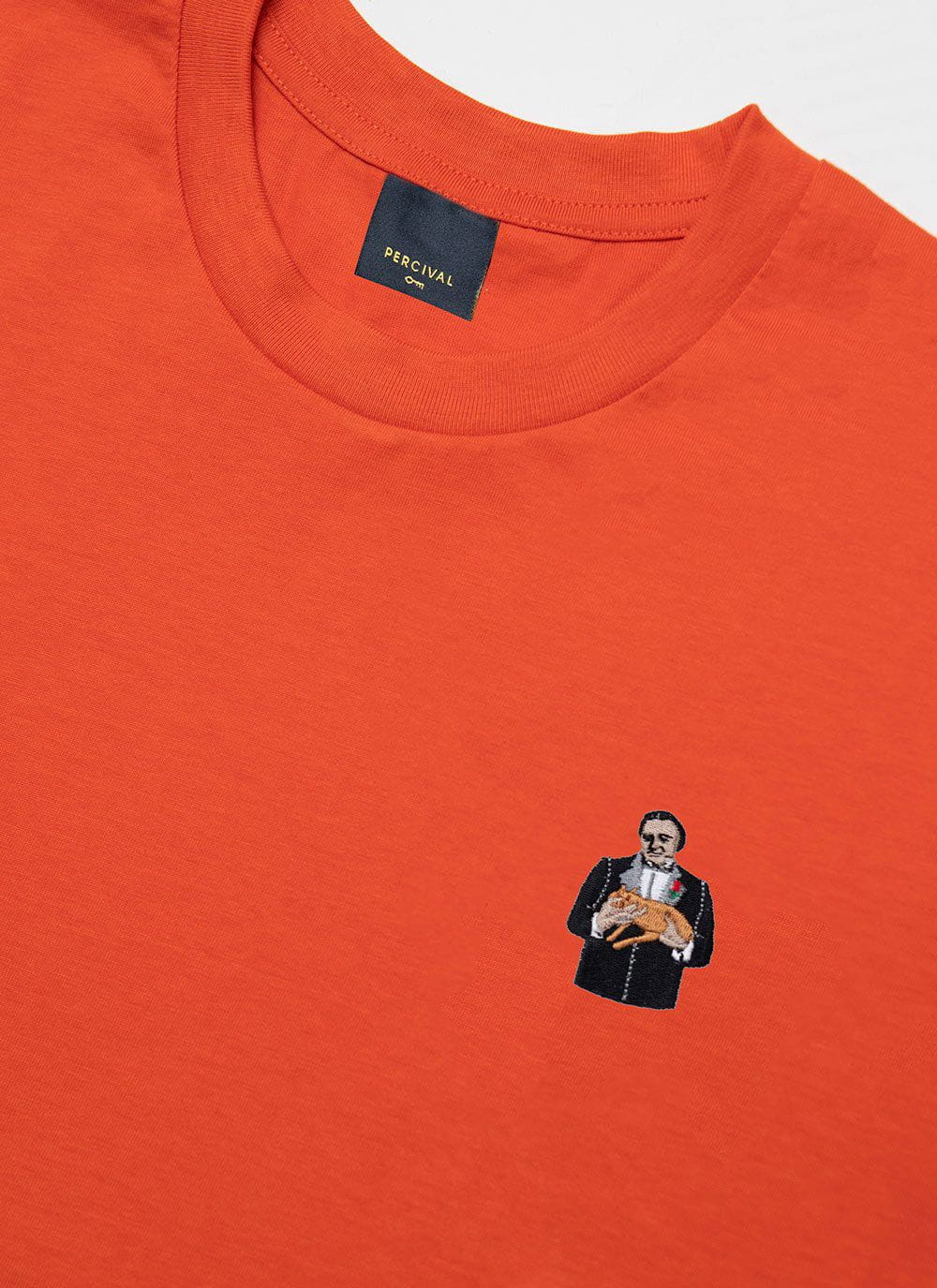• XS
• S
• M
• L
• XL
• XXL
• Select Size
Running Low• XS
• S
• M
• L
• XL
• XXL
• Select Size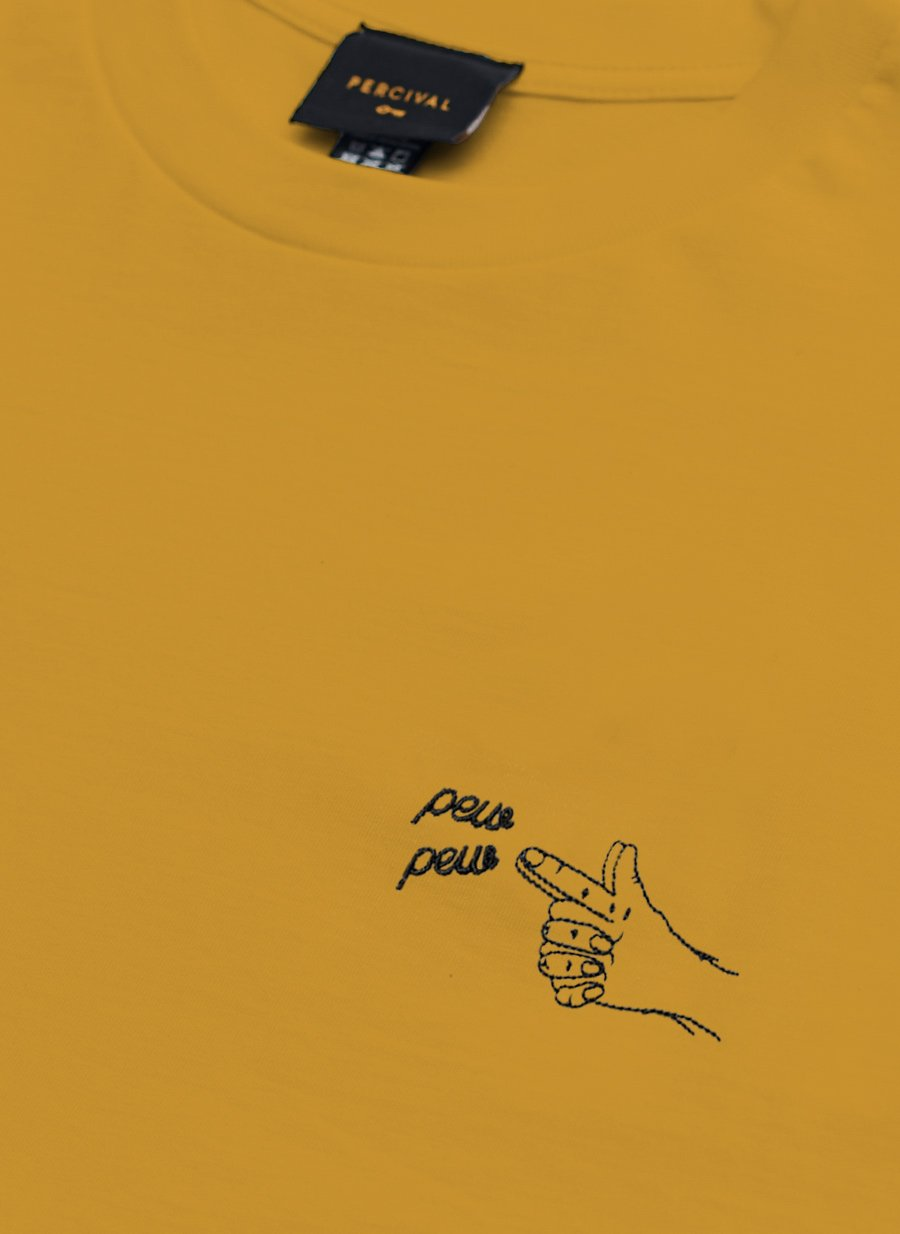• XS
• S
• M
• L
• XL
• XXL
• Select Size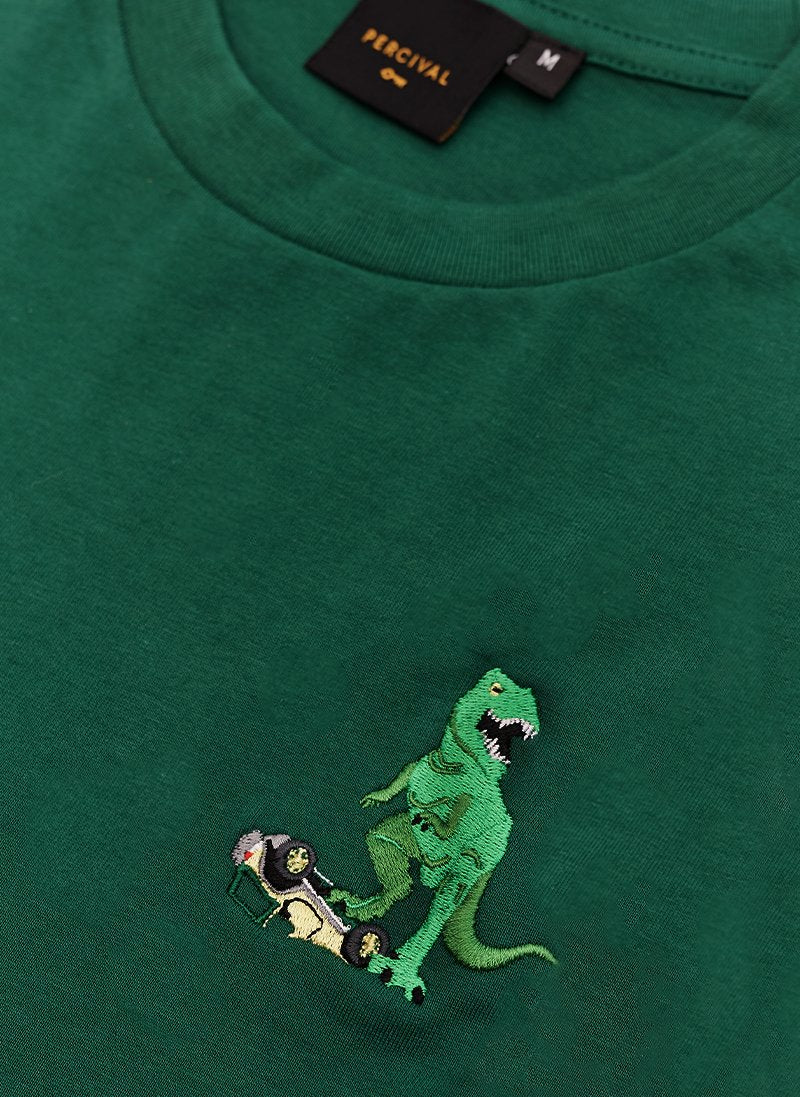• XS
• S
• M
• L
• XL
• XXL
• Select Size• XS
• S
• M
• L
• XL
• XXL
• Select Size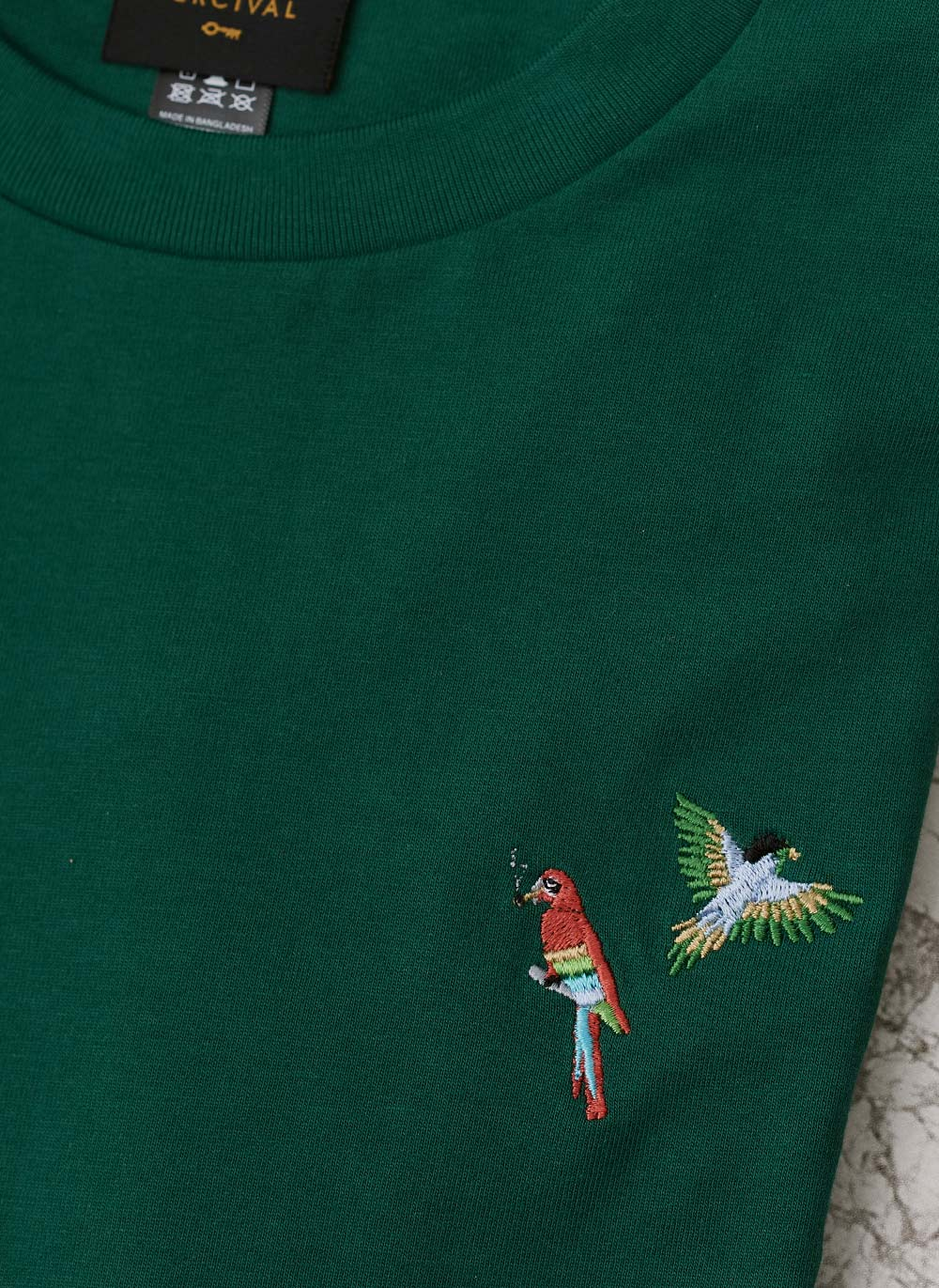• XS
• S
• M
• L
• XL
• XXL
• Select Size